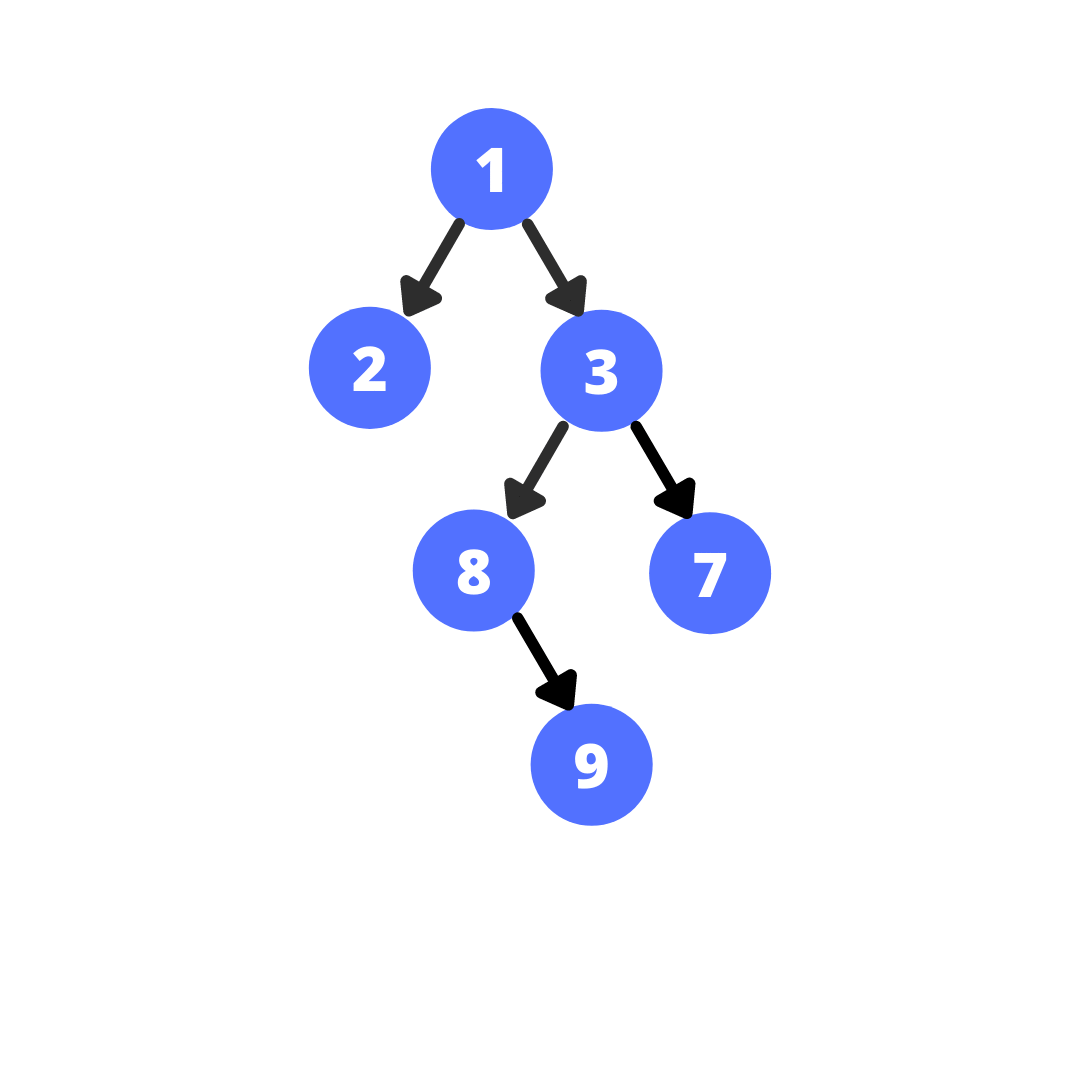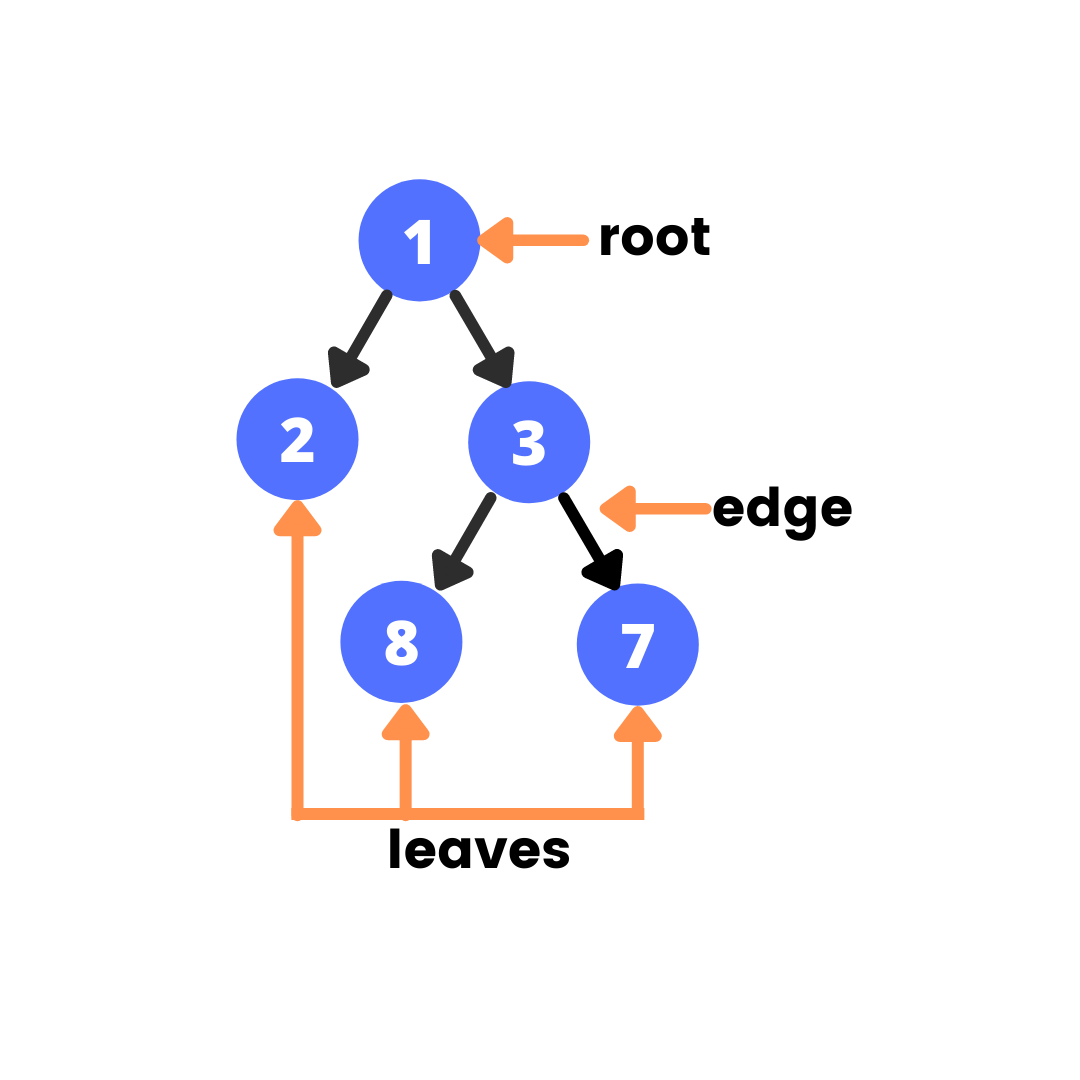# Introduction to Data Structures - Tree

##### Trees are one of the non-linear data structures used to represent or store hierarchal data.Batoi Research Group Apr 1, 2021

#### What Are Trees?

Trees are one of the non-linear data structures used to represent or store hierarchical data. Trees are a collection of nodes, with every node holding some value and a reference to multiple nodes called children nodes.

Terminologies in Tree:

• Each node is connected to its parent and child nodes with edges.
• The topmost node in the hierarchy is called the root node.
• Not all the nodes are required to have children. The nodes with zero child nodes are called leaf nodes.
• The height of the tree is given by the longest path from the root node to the leaf node.
• The depth of a node in a tree is its distance from the root node.

#### Where Do We Use Trees?

Trees can store hierarchical data such as folder structures and are also used in the HTML Document Object Model (DOM).

#### Representation of Trees

We create a custom data type TreeNode that holds the value and pointer to its child nodes as its members to represent a tree. A generic tree, also known as n-ary trees, permits each node to have n children.

The most fundamental Tree is known as binary trees, where every node can have a maximum of two children. Moving further we can have ternary trees with three children and so on.

#### Binary Trees

As we now know binary trees are trees with a maximum of two child nodes called left child and right child. Let’s see how we can define one:

class TreeNode{        int val;    TreeNode* left;    TreeNode* right;    TreeNode(int data){        val = data;        left = NULL;        right = NULL;    }};

#### Tree Traversal

A Tree traversal or tree search is the method of visiting/checking each node of the tree exactly once. There are two ways to search a tree:

2. Depth First Search

BFS is a method of searching the tree breadth wise meaning visiting all the nodes at one level before moving on to the next level. Let’s say we have the following tree:A Breadth-first traversal of this tree will give [1,2,3,8,7,9] as output.

void BFS(TreeNode* root){    queue Q;    Q.push(root);    while(!Q.empty()){        TreeNode* front = Q.front();        Q.pop();        cout<val<<" ";        if(front->left) Q.push(front->left);        if(front->right) Q.push(front->right);    }}

#### Depth First Search (DFS)

DFS is a method of searching the tree where we start at the root node and explore all the nodes and the depth of the current path and then backtrack to explore another approach.

There are three types of Depth-first searching in binary trees:

• Pre-order traversal
• In-order traversal
• Post-order traversal

#### Pre-order Traversal

In pre-order, the data of the root node is accessed first. After that, the data in the left-sub tree is accessed, and the data of the right-subtree is accessed last. The traversal is recursive.

void preorder(TreeNode* root){    if(!root) return;    cout<val;    preorder (root->left);    preorder (root->right);}The in-order traversal of this tree will give [1,2,3,8,7] as output.

#### In-order Traversal

In in-order, the data in the left-sub tree is accessed first. After that, the data of the root node is accessed, and the data of the right-subtree is accessed last. The traversal is recursive.

void inorder(TreeNode* root){    if(!root) return;    inorder(root->left);    cout<val;    inorder(root->right);}The in-order traversal of this tree will give [2,1,8,3,7] as output.

#### Post-order Traversal

In post-order, data in the left-sub tree is accessed first. After that, the data of the right-subtree is accessed, and the root node data is accessed at last. The traversal is recursive.

void postorder(TreeNode* root){    if(!root) return;    postorder (root->left);    postorder (root->right);    cout<val;}The post-order traversal of this tree will give [2,8,7,3,1] as output

#### Important Binary Tree Concepts

Strictly/Full Binary Tree: A Binary tree is called a full binary tree if all the nodes have 0 or 2 children.

Complete Binary Tree: A complete binary tree is a tree where all the levels are filled except the last one. And all the leaves in the last level lean towards the left as much as possible.

Balanced Tree: A tree is called balanced if the height of the left-subtree and height of the right-subtree differs by at most 1.

#### Special Types of Trees

Binary Search Trees: BST is trees in which all the nodes in the left subtree are smaller than the root node, and all the nodes in the right subtree are more significant than the root node.
Usage:

• It can be used to implement a sorting algorithm.
• It can be used to implement a priority queue.

Self-balancing Trees: These types of trees have a unique property that the tree balances itself every time a data is inserted or deleted.

Heap Trees: Heap trees are the trees that keep the node with the highest priority as the root node of the tree.

### Need our assistance? We are available with you.